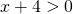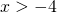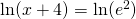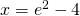# Solution assignment 01 Logarithmic equations

### Assignment 1

Solve:### Solution

The equation is defined if, so if(the domain).
We can write the equation as:yielding:This solution is in the domain and thus is a valid solution.